×#### Thank you for registering.

One of our academic counsellors will contact you within 1 working day.

Click to Chat

1800-1023-196

+91-120-4616500

CART 0

• 0

MY CART (5)

Use Coupon: CART20 and get 20% off on all online Study Material

ITEM
DETAILS
MRP
DISCOUNT
FINAL PRICE
Total Price: Rs.

There are no items in this cart.
Continue Shopping• Complete JEE Main/Advanced Course and Test Series
• OFFERED PRICE: Rs. 15,900
• View Details

```Chapter 10: Circles Exercise – 10.2

Question: 1

If PT is a tangent at T to a circle whose center is 0 and OP = 17 cm, OT = 8 cm. Find the length of tangent segment PT.

Solution:

OT = radius = 8 cm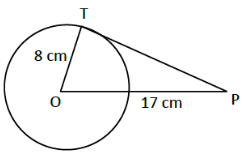OP = 17 cm

PT = length of tangent =?

T is point of contact. We know that at point of contact tangent and radius are perpendicular.

∴ OTP is right angled triangle ∠OTP = 90°, from Pythagoras theorem OT2 + PT2 = OP2

82 + PT2 = 172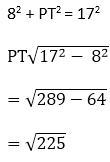∴ PT = length of tangent = 15 cm.

Question: 2

Find the length of a tangent drawn to a circle with radius 5cm, from a point 13 cm from the center of the circle.

Solution:

Consider a circle with center O.

OP = radius = 5 cm.

A tangent is drawn at point P, such that line through O intersects it at Q, OB = 13cm.

Length of tangent PQ =?A + P, we know that tangent and radius are perpendicular.

∆OPQ is right angled triangle, ∠OPQ = 90°

By Pythagoras theorem, OQ2 = OP2 + PQ2

⇒ 132 = 52 + PQ2

⇒ PQ2 = 169 - 25 = 144

⇒ PQ = √114

= 12 cm

Length of tangent = 12 cm

Question: 3

A point P is 26 cm away from 0 of circle and the length PT of the tangent drawn from P to the circle is 10 cm. Find the radius of the circle.

Solution:

Given OP = 26 cm

PT = length of tangent = 10 cm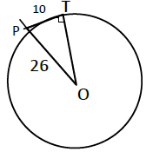At point of contact, radius and tangent are perpendicular ∠OTP = 90°, ∆OTP is right angled triangle. By Pythagoras theorem,

OP2 = OT2 + PT2

262 = OT2 + 102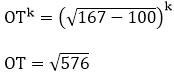= 24 cm

OT = length of tangent = 24 cm

Question: 4

If from any point on the common chord of two intersecting circles, tangents be drawn to circles, prove that they are equal.

Solution:

Let the two circles intersect at points X and Y.

XY is the common chord.

Suppose 'A' is a point on the common chord and AM and AN be the tangents drawn A to the circle

We need to show that AM = AN.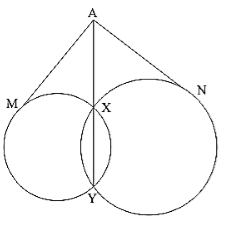In order to prove the above relation, following property will be used.

"Let PT be a. tangent to the circle from an external point P and a secant to the circle through P intersects the circle at points A and B, then PT2 = PA × PB"

Now AM is the tangent and AXY is a secant ∴ AM2 = AX × AY ... (i)

AN is a tangent and AXY is a secant

∴ AN2 = AX × AY .... (ii)

From (i) & (ii), we have AM2 = AN2

∴ AM = AN

Question: 5

If the quadrilateral sides touch the circle prove that sum of pair of opposite sides is equal to the sum of other pair.

Solution:

Consider a quadrilateral ABCD touching circle with center O at points E, F, G and 11 as in figure.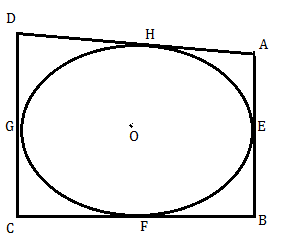We know that

The tangents drawn from same external points to the circle are equal in length.

1. Consider tangents from point A [AM ⊥ AE]

AH = AE ... (i)

2. From point B [EB & BF]

BF = EB ... (ii)

3. From point C [CF & GC]

FC = CG ... (iii)

4. From point D [DG & DH]

DH = DG .... (iv)

Adding (i), (ii), (iii), & (iv)

(AH + BF + FC + DH) = [(AC + CB) + (CG + DG)]

⟹ (AH + DH) + (BF + FC) = (AE + EB) + (CG + DG)

⟹ AD + BC = AB + DC [from fig.]

Sum of one pair of opposite sides is equal to other.

Question: 6

If AB, AC, PQ are tangents in Fig. and AB = 5 cm find the perimeter of ∆APQ.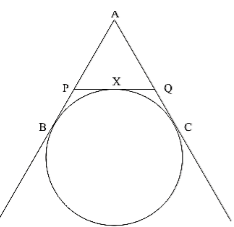Solution:

Perimeter of AAPQ, (P) = AP + AQ + PQ

= AP + AQ + (PX + QX)We know that

The two tangents drawn from external point to the circle are equal in length from point A,

AB = AC = 5 cm

From point P, PX = PB

From point Q, QX = QC

Perimeter (P) = AP + AQ + (PB + QC)

= (AP + PB) + (AQ + QC)

= AB + AC = 5 + 5

= 10 cm.

Question: 7

Prove that the intercept of a tangent between two parallel tangents to a circle subtends a right angle at center.

Solution:

Consider circle with center 'O' and has two parallel tangents through A & B at ends of diameter.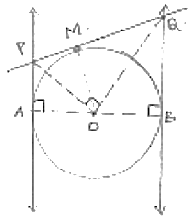Let tangents through M intersects the tangents parallel at P and Q required to prove is that LPOQ = 90°. From fig. it is clear that ABQP is a quadrilateral

∠A + ∠B = 90° + 90° = 180° [At point of contact tangent & radius are perpendicular]

∠A + ∠B + ∠P + ∠Q = 360° [Angle sum property]

∠P + ∠Q = 360° - 180° = 180° ... (i)

At P & Q ∠APO = ∠OPQ =1/2 ∠P

∠BQO = ∠PQO = 1/2 ∠Q    in (i)

2∠OPQ + 2∠PQO = 180°

∠OPQ + ∠PQO = 90° ...  (ii)

In ∆OPQ, ∠OPQ + ∠PQO + ∠POQ = 180° [Angle sum property]

90° + ∠POQ = 180°           [from (ii)]

∠POQ = 180° – 90° = 90°

∠POQ = 90°

Question: 8

In Fig below, PQ is tangent at point R of the circle with center O. If ∠TRQ = 30°. Find LPRS.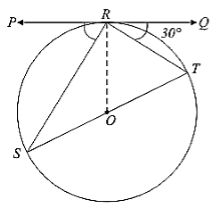Solution:

Given ∠TRQ = 30°.

At point R, OR ⊥ RQ.

∠ORQ = 90°

⟹ ∠TRQ + LORT = 90°

⟹ ∠ORT = 90°- 30° = 60°

ST is diameter, LSRT = 90° [ ∵ Angle in semicircle = 90°]

∠ORT + ∠SRO = 90°

∠SRO + ∠PRS = 90°

∠PRS = 90°- 30° = 60°

Question: 9

If PA and PB are tangents from an outside point P. such that PA = 10 cm and LAPB = 60°. Find the length of chord AB.

Solution:

AP = 10 cm ∠APB = 60°

Represented in the figureWe know that

A line drawn from center to point from where external tangents are drawn divides or bisects the angle made by tangents at that point ∠APO = ∠OPB =1/2 × 60° = 30°

The chord AB will be bisected perpendicularly

∴ AB = 2AM

In ∆AMP,AM = AP sin 30°

AP/2 = 10/2 = 5cm

AP = 2 AM =10 cm ... Method (i)

In ∆AMP, ∠AMP = 90°, ∠APM = 30°

∠AMP + ∠APM + ∠MAP = 180°

90° + 30° + ∠MAP = 180°

∠MAP = 180°

In ∆PAB, ∠MAP = ∠BAP = 60°, ∠APB = 60°

We also get, ∠PBA = 60°

∴ ∆PAB is equilateral triangle

AB = AP = 10 cm ... Method (ii)
```### Course Features

• 728 Video Lectures
• Revision Notes
• Previous Year Papers
• Mind Map
• Study Planner
• NCERT Solutions
• Discussion Forum
• Test paper with Video Solution